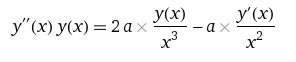## Recommended Posts

Is it possible to find an analytical solution to the following differential equationThank you in advance to those who will respond

##### Share on other sites
Posted (edited)

This could be a start.

If I have read you correctly, then I have separated the variables for you.

Edit, Whoopsy I have needed to edit last line as can be seen.

Sorry.

Edited by studiot

##### Share on other sites
Posted (edited)

By inspection, y=0 is solution.

Now, after a couple of tries, one gets the intuition that the solution is some kind of potential function, so let's try that out:

$y=kx^{p}$

then,

$y'=pkx^{p-1}$

$y''=p\left(p-1\right)kx^{p-2}$

Substituting and finding condition for p and k:

$k^{2}p\left(p-1\right)x^{2p-2}=ak\left(2-p\right)x^{p-3}$

$\Rightarrow p=-1$

$2k^{2}=ak\Rightarrow$

$k=0$

(the trivial solution we found by inspection) or:

$k=\frac{a}{2}$

with which,

$y=\frac{a}{2}x^{-1}$

Mind you, non-linear differential equations may have more families* of solutions. Check me for mistakes.

* Typically, not families. Rather, isolated solutions.

Edited by joigus

##### Share on other sites
1 hour ago, joigus said:

Now, after a couple of tries, one gets the intuition that the solution is some kind of potential function, so let's try that out:

Thank you.

Yes, this is the equation for a point mass field, taking into account the mass of the field itself.

I used to think the density of the gravitational field was equal ro=g^2/4*Pi*c^2 which led to an incorrect decision.

Replacing c^2 with phi led to an equation that I asked for help solving. In him y(x)=phi(r) and a=4*Gm.

The solution you found gives Newton's law of gravity

phi(r) = -2Gm/r g(r)=(dPhi/dr)/2=-Gm/r^2

It turns out that taking into account the mass of the gravitational field itself does not change anything in Newton's law of gravity.

##### Share on other sites
5 minutes ago, SergUpstart said:

Thank you.

Yes, this is the equation for a point mass field, taking into account the mass of the field itself.

I used to think the density of the gravitational field was equal ro=g^2/4*Pi*c^2 which led to an incorrect decision.

Replacing c^2 with phi led to an equation that I asked for help solving. In him y(x)=phi(r) and a=4*Gm.

The solution you found gives Newton's law of gravity

phi(r) = -2Gm/r g(r)=(dPhi/dr)/2=-Gm/r^2

It turns out that taking into account the mass of the gravitational field itself does not change anything in Newton's law of gravity.

So you were thinking about gravitational potentials from the get go, and you couldn't figure out that a plausible solution was k/x? Something doesn't add up here. This sounds a bit disingenuous... Is it gravity you want to talk about, instead of calculus?

##### Share on other sites
Posted (edited)
5 hours ago, joigus said:

So you were thinking about gravitational potentials from the get go, and you couldn't figure out that a plausible solution was k/x? Something doesn't add up here. This sounds a bit disingenuous... Is it gravity you want to talk about, instead of calculus?

No, I really couldn't solve this equation for a long time and decided to turn to mathematicians. How could I have known that you would solve the equation? I was hoping that the solution would converge to Gm/r^2 in the asymptotic for large r, but not exactly match it.

Edited by SergUpstart

##### Share on other sites
Posted (edited)

10 hours ago, joigus said:

Now, after a couple of tries, one gets the intuition that the solution is some kind of potential function, so let's try that out:

y=kxp

An exponential function is not suprising since my last line leads to three integrals two of which are standard logarithmic ones.
The third one need to be handled by parts.

So it is one of those situations where you have multiple competing functions and  it depends (as you note) on aditional information (boundary conditions) which dominates.

+1 to joigus for 'the physicist's method'

Edited by studiot

##### Share on other sites
Posted (edited)
9 hours ago, SergUpstart said:

Yes, this is the equation for a point mass field, taking into account the mass of the field itself.

In Newtonian physics, gravity is a linear interaction - meaning the field does not self-interact, so it is not possible to attribute mass to it. One could thus have guessed at the end result without needing to solve this (really awkward) equation.
For a model of gravity that is non-linear, i.e. where the field self-interacts, have a look at General Relativity as well as it numerous off-shoots and alternatives.

Edited by Markus Hanke

##### Share on other sites
7 hours ago, Markus Hanke said:

In Newtonian physics, gravity is a linear interaction - meaning the field does not self-interact, so it is not possible to attribute mass to it. One could thus have guessed at the end result without needing to solve this (really awkward) equation.
For a model of gravity that is non-linear, i.e. where the field self-interacts, have a look at General Relativity as well as it numerous off-shoots and alternatives.

I've missed that point. +1

I was so distracted with the equation itself, and then the OP completely changed the subject to gravity. It threw me off...

## Create an account

Register a new account